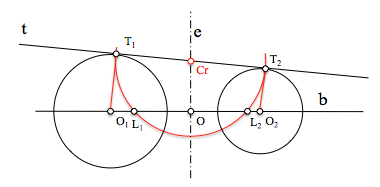# Metric geometry : Generalization of the fundamental problem of tangents :We have resolved that we call fundamental problem of tangents when presented with tangency conditions of a circle or a straight. Conceptually we can assume that both problems are the same, if we consider the straight as a circle of infinite radius. The statement raised thus obtaining circumferences passing through two points were tangent to a straight or tangential to a circumference.

In both cases therefore apply similar reasoning for resolution, based on the concepts learned in power.

Considering that circles through two points belong to a elliptical beam circumferences, We can generalize the fundamental problem of tangents (PFT) enunciating follows:

Determining the circumferences of a corradicales beam circumferences which are tangent to a geometric element (line the circumference)

We solved these problems by studying separately each type of beam:

In all three cases we have analyzed the case in which the condition of tangency is a line or circle.Hyperbolic tangent circles of a straight beam

The solution is to determine a point of equal power, Cr, regarding the condition of tangency and with respect to which the beam belongs solution. If the condition is compared to a straight, searched point is at the intersection of this line with the radical axis.If the tangency condition is with respect to a circle we also locate the point of equal power with respect to the beam and the circumference, for which we obtain an auxiliary radical axis (e2) between the tangency condition and any circumference of the beam.

The power of this point, Cr, regarding the condition of tangency determine the points of contact between the circumference and the solutions belonging to the beam.

Metric geometry